# # 在 Azure ML 上使用生成对抗网络（GAN）创建艺术作品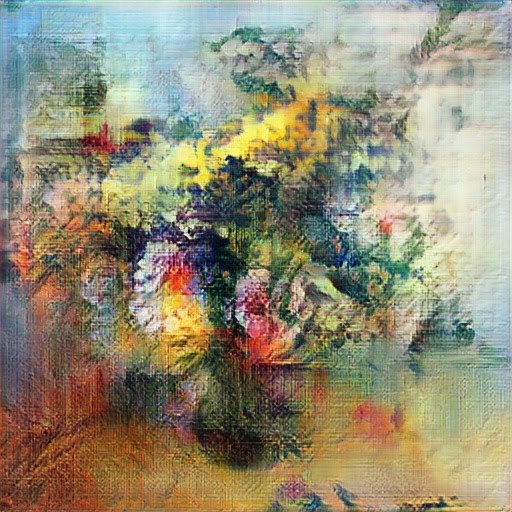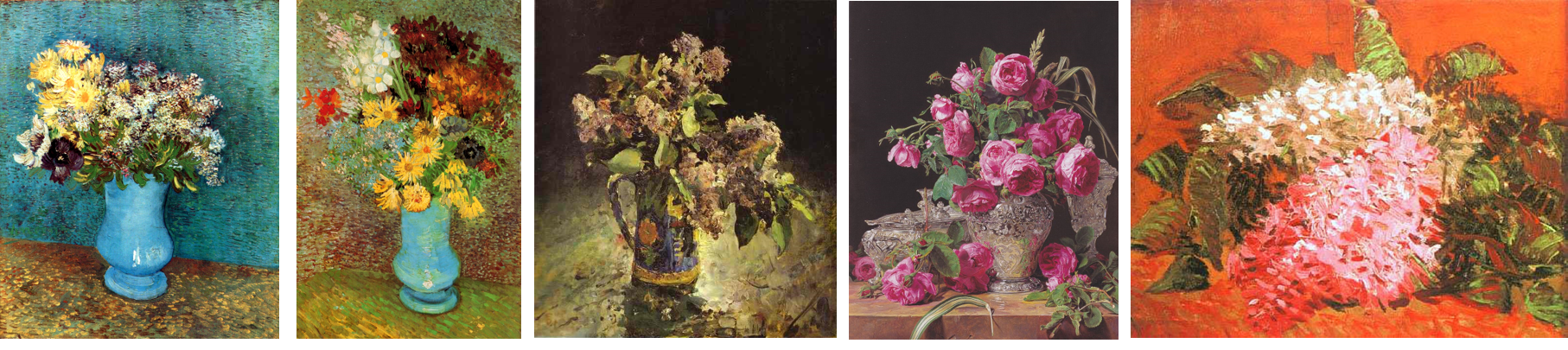## # 生成对抗网络

GAN由两个网络组成：

• 生成器, 根据给定的输入噪声矢量生成图片
• 识别器, 区分真实绘画和“假”（生成的）绘画的不同点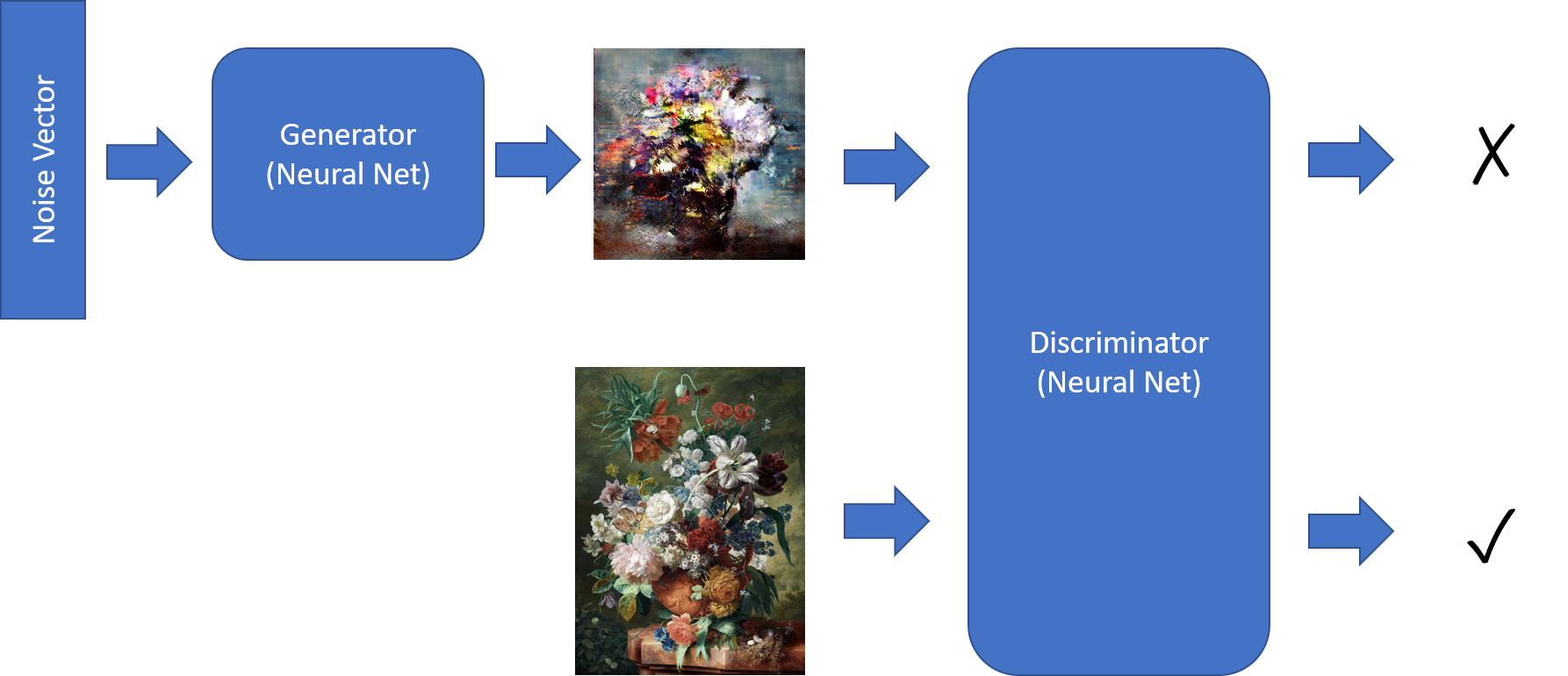1. 获取一堆生成的和真实的图片：

``````noise = np.random.normal(0, 1, (batch_size, latent_dim))
gen_imgs = generator.predict(noise)
imgs = get_batch(batch_size)
``````
2. 训练识别器以更好的区分两者。注意：我们是提供向量值的方法`ones``zeros`

``````d_loss_r = discriminator.train_on_batch(imgs, ones)
d_loss_f = discriminator.train_on_batch(gen_imgs, zeros)
``````
1. 训练组合模型，以提升生成器

``````g_loss = combined.train_on_batch(noise, ones)
``````

``````discriminator = create_discriminator()
generator = create_generator()
discriminator.compile(loss='binary_crossentropy', optimizer=optimizer,
metrics=['accuracy'])
discriminator.trainable = False

z = keras.models.Input(shape=(latent_dim,))
img = generator(z)
valid = discriminator(img)

combined = keras.models.Model(z, valid)
combined.compile(loss='binary_crossentropy', optimizer=optimizer)
``````

## # 识别器模型

``````discriminator = Sequential()

for x in [16,32,64]: # number of filters on next layer

``````

• 形状为64x64x3的原始图像经过16层过滤器，产生一个形状为32x32x16的图像。为了减小文件大小，我们使用 `AveragePooling2D`
• 下一步转换32x32x16的张量为16x16x32的张量。
• 最后，在下一个卷积层之后，我们最终得到8x8x64形状的张量。

## # 生成器模型

``````generator = Sequential()
generator.add(Dense(8 * 8 * 2 * size, activation="relu",
input_dim=latent_dim))

for x in [64;32;16]:

``````

## # Azure ML的训练脚本

Azure ML除了支持记录数值之外还支持记录图像，具体请看此处。我们可以记录呈现为np数组的图像，或者是由`matplotlib`生成的任何图表，因此我们将在一个图表上绘制三个示例图像。这个绘图过程将在每次训练之后由`keragan`调用的回调函数`callbk`中处理：

``````def callbk(tr):
if tr.gan.epoch % 20 == 0:
res = tr.gan.sample_images(n=3)
fig,ax = plt.subplots(1,len(res))
for i,v in enumerate(res):
ax[i].imshow(v)
run.log_image("Sample",plot=plt)
``````

``````gan = keragan.DCGAN(args)
imsrc = keragan.ImageDataset(args)
train = keragan.GANTrainer(image_dataset=imsrc,gan=gan,args=args)

train.train(callbk)
``````

## # 开始实验

• 使用 `ws = Workspace.from_config()`连接到工作空间
• 连接到计算集群： `cluster = ComputeTarget(workspace=ws, name='My Cluster')`。此处我们需要一个带有GPU的虚拟机集群，例如NC6
• 上传我们的数据集到ML工作区默认的数据存储里

``````exp = Experiment(workspace=ws, name='KeraGAN')
script_params = {
'--path': ws.get_default_datastore(),
'--dataset' : 'faces',
'--model_path' : './outputs/models',
'--samples_path' : './outputs/samples',
'--batch_size' : 32,
'--size' : 512,
'--learning_rate': 0.0001,
'--epochs' : 10000
}
est = TensorFlow(source_directory='.',
script_params=script_params,
compute_target=cluster,
entry_script='train_gan.py',
use_gpu = True,
conda_packages=['keras','tensorflow','opencv','tqdm','matplotlib'],
pip_packages=['git+https://github.com/shwars/keragan@v0.0.1']

run = exp.submit(est)
``````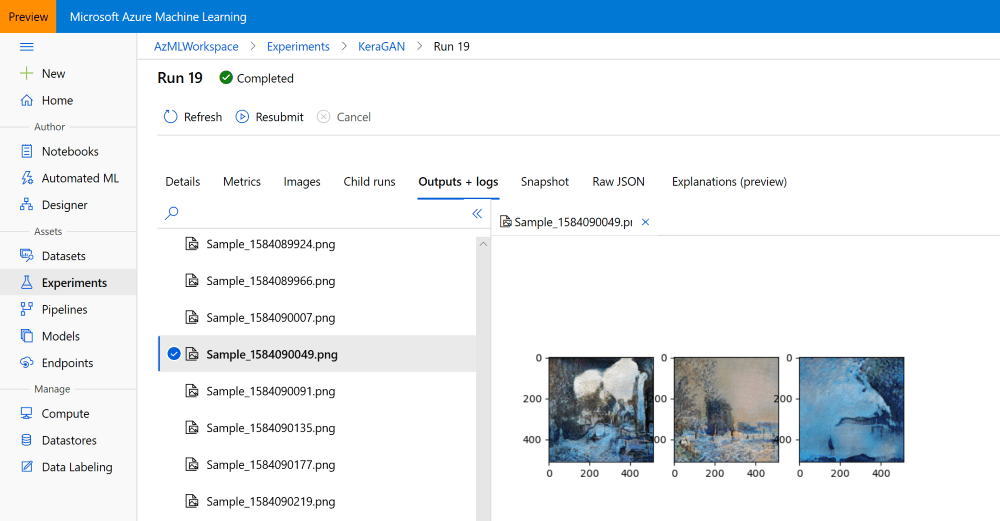## # 运行多个实验

• `--大小` 这决定了图片的大小，数值应该是2的指数级。像64或者128这样的小型号可以让实验更迅速，然而大型号（最高可达1024）有利于生成高质量的图片。超过1024可能就无法产生一个好的结果，因为高分辨率的GANs需要特殊的技术来训练，比如 progressive growing
• `--学习速率` 是一个（令人惊讶的）相当主要的参数，尤其是对于高分辨率图像的训练。学习速率越小，训练的结果就越好，但同时也会非常的慢。
• `--数据集` 我们可能会上传不同风格的图片到Azure ML数据存储中的不同文件夹里面，并同时开始训练多个实验。

## # 获得实验结果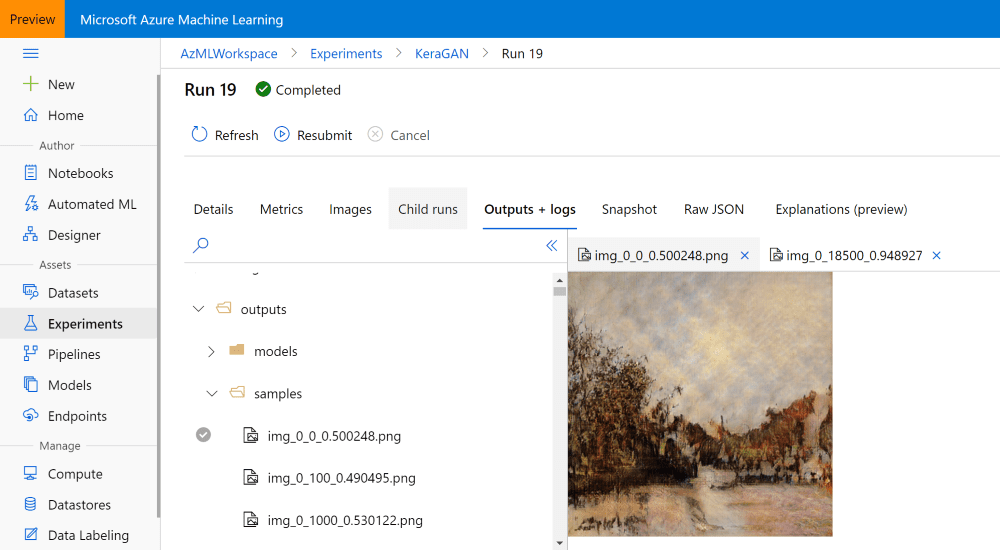``````run.download_files(prefix='outputs/samples')
``````

``````run = Run(experiment=exp,run_id='KeraGAN_1584082108_356cf603')
``````

``````fnames = run.get_file_names()
fnames = filter(lambda x : x.startswith('outputs/models/gen_'),fnames)
``````

``````no = max(map(lambda x: int(x[19:x.find('.')]), fnames))
fname = 'outputs/models/gen_{}.h5'.format(no)
fname_wout_path = fname[fname.rfind('/')+1:]
``````

## # 生成新图像

``````model = keras.models.load_model(fname_wout_path)
latent_dim=model.layers.input.shape.value
res = model.predict(np.random.normal(0,1,(10,latent_dim)))
``````

``````res = (res+1.0)/2
fig,ax = plt.subplots(1,10,figsize=(15,10))
for i in range(10):
ax[i].imshow(res[i])
``````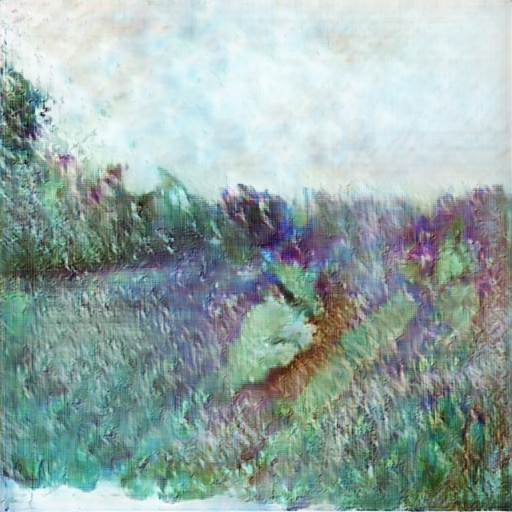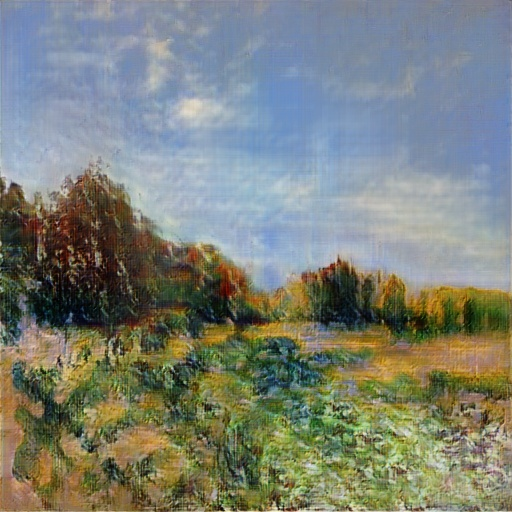keragan 基于 WikiArt 印象派的训练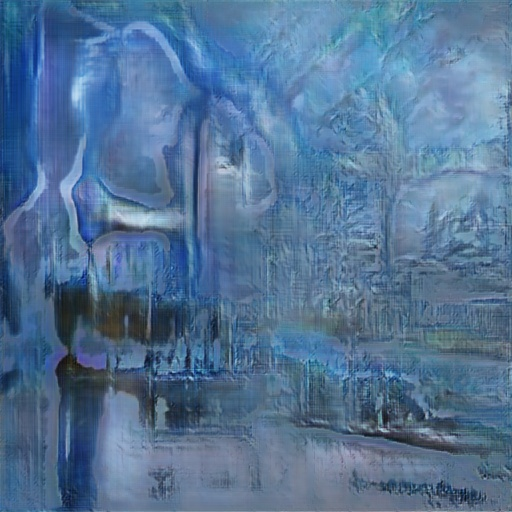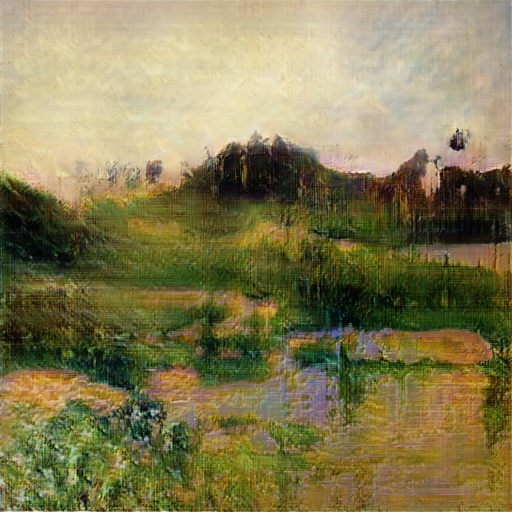keragan 基于 WikiArt 印象派的训练

## # 观察学习的过程

GAN Flower Generation GAN Portrait Generation
https://youtu.be/hnwbnt2Q9Iw https://youtu.be/j2wpUFxyrEs

##致谢